International
Tables for
Crystallography
Volume F
Crystallography of biological macromolecules
Edited by M. G. Rossmann and E. Arnold

International Tables for Crystallography (2006). Vol. F, ch. 11.5, pp. 236-237   | 1 | 2 |

Section 11.5.2. Generalization of the Hamilton, Rollett and Sparks equations to take into account partial reflections

C. G. van Beek,a R. Bolotovskya§ and M. G. Rossmanna*

aDepartment of Biological Sciences, Purdue University, West Lafayette, IN 47907-1392, USA
Correspondence e-mail:  mgr@indiana.bio.purdue.edu

11.5.2. Generalization of the Hamilton, Rollett and Sparks equations to take into account partial reflections

| top | pdf |

When a Bragg reflection is completely exposed within the oscillation range of one frame, a so-called full reflection', it gives rise to the full intensity'. In general, a Bragg reflection will occur on a number of consecutive frames as a series of partial reflections, and the full intensity can only be estimated from the measured intensities of the partial reflections. Let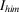represent the intensity contribution of reflectionrecorded on frame m; if all the parts ofare available in the data set, then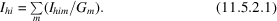In practice, there will always be reflections that do not have all their parts available. In such cases, the only way to estimate the full intensity of a reflection is to apply an estimated value of the partiality to the measured reflection intensities.

Various models have been proposed to calculate the reflection partiality. Here we use Rossmann's model (Rossmann, 1979; Rossmann et al., 1979) with Greenhough & Helliwell's (1982) correction. This model treats partiality as a fraction of a spherical volume swept through the Ewald sphere. The coordinates of the reciprocal-lattice point are defined by the Miller indices of the reflection, the crystal orientation matrix and the rotation angle. The volume of the sphere around the reciprocal-lattice point accounts for crystal mosaicity and beam divergence. Alternative geometrical descriptions of a reciprocal-lattice point passing through the Ewald sphere have been given by Winkler et al. (1979) and Bolotovsky & Coppens (1997).

Provided the reflection partiality,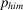, is known, the full intensity is estimated by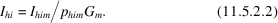This expression can produce as many estimates ofas there are parts of reflection, while expression (11.5.2.1) produces only one estimate ofwhen all parts of reflectionare recorded. Having defined the relationships between measured intensities of partial reflections and estimated full reflections by expressions (11.5.2.1) and (11.5.2.2), two methods of generalizing the HRS equations can be considered.

 Method 1. If a reflectionoccurs on a number of consecutive frames and all parts ofare available in the data set, the generalized HRS target equation takes the form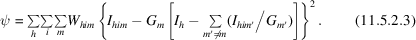Using expression (11.5.1.2), the best least-squares estimate ofwill be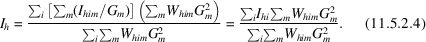Method 2. If the theoretical partiality,, of the partially recorded reflection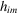can be estimated, the generalized HRS target equation takes the form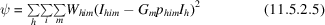and, using expression (11.5.1.2), the best least-squares estimate ofwill then be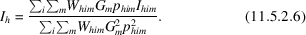When all reflections in the data set are fully recorded, expressions (11.5.2.3) and (11.5.2.5) reduce to the `classical' HRS expression (11.5.1.1), and expressions (11.5.2.4) and (11.5.2.6) reduce to expression (11.5.1.3).

The scale factor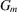can be generalized to incorporate crystal decay (Gewirth, 1996; Otwinowski & Minor, 1997):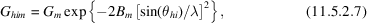where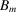is a parameter describing the crystal disorder while frame m was recorded,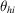is the Bragg angle of reflectionand λ is the X-ray wavelength.

Method 1only allows the refinement of the scale factors while method 2allows refinement of the scale factors, crystal mosaicity and orientation matrix, as the latter two factors contribute to the calculated partiality. Furthermore, method 2is essential for scaling of data sets with low redundancy (e.g. data collected from low-symmetry crystals or data collected over small rotation ranges). When a reflectionspans more than one frame, but there are no other reflections with the same reduced Miller indices h in the data set, the contribution of any partial reflectionto expression (11.5.2.3) will be zero, as in this casewill be the same as. In contrast, in method 2the reflectioncan be used for scaling because the estimates of the full intensityare calculated independently from every frame spanned by reflection.

References

Bolotovsky, R. & Coppens, P. (1997). The ϕ extent of the reflection range in the oscillation method according to the mosaicity-cap model. J. Appl. Cryst. 30, 65–70.Google Scholar
Gewirth, D. (1996). The HKL manual. A description of the programs DENZO, XDSPLAYF and SCALEPACK, 5th ed., pp. 87–90. Yale University, New Haven, USA.Google Scholar
Greenhough, T. J. & Helliwell, J. R. (1982). Oscillation camera data processing: reflecting range and prediction of partiality. I. Conventional X-ray sources. J. Appl. Cryst. 15, 338–351.Google Scholar
Otwinowski, Z. & Minor, W. (1997). Processing of X-ray diffraction data collected in oscillation mode. Methods Enzymol. 276, 307–326.Google Scholar
Rossmann, M. G. (1979). Processing oscillation diffraction data for very large unit cells with an automatic convolution technique and profile fitting. J. Appl. Cryst. 12, 225–238.Google Scholar
Rossmann, M. G., Leslie, A. G. W., Abdel-Meguid, S. S. & Tsukihara, T. (1979). Processing and post-refinement of oscillation camera data. J. Appl. Cryst. 12, 570–581.Google Scholar
Winkler, F. K., Schutt, C. E. & Harrison, S. C. (1979). The oscillation method for crystals with very large unit cells. Acta Cryst. A35, 901–911.Google Scholar